# 1.4 Polynomials  (Page 3/15)

 Page 3 / 15

Find the product.

$\left(3x+2\right)\left({x}^{3}-4{x}^{2}+7\right)$

$3{x}^{4}-10{x}^{3}-8{x}^{2}+21x+14$

## Using foil to multiply binomials

A shortcut called FOIL is sometimes used to find the product of two binomials. It is called FOIL because we multiply the f irst terms, the o uter terms, the i nner terms, and then the l ast terms of each binomial.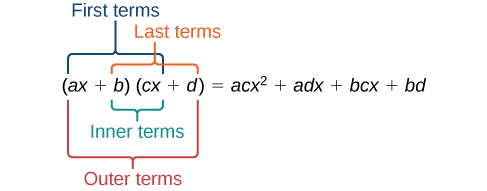The FOIL method arises out of the distributive property. We are simply multiplying each term of the first binomial by each term of the second binomial, and then combining like terms.

Given two binomials, use FOIL to simplify the expression.

1. Multiply the first terms of each binomial.
2. Multiply the outer terms of the binomials.
3. Multiply the inner terms of the binomials.
4. Multiply the last terms of each binomial.
6. Combine like terms and simplify.

## Using foil to multiply binomials

Use FOIL to find the product.

$\left(2x-18\right)\left(3x+3\right)$

Find the product of the first terms.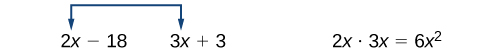Find the product of the outer terms.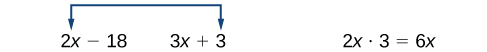Find the product of the inner terms.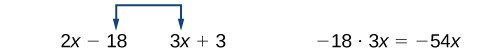Find the product of the last terms.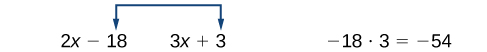Use FOIL to find the product.

$\left(x+7\right)\left(3x-5\right)$

$3{x}^{2}+16x-35$

## Perfect square trinomials

Certain binomial products have special forms. When a binomial is squared, the result is called a perfect square trinomial    . We can find the square by multiplying the binomial by itself. However, there is a special form that each of these perfect square trinomials takes, and memorizing the form makes squaring binomials much easier and faster. Let’s look at a few perfect square trinomials to familiarize ourselves with the form.

Notice that the first term of each trinomial is the square of the first term of the binomial and, similarly, the last term of each trinomial is the square of the last term of the binomial. The middle term is double the product of the two terms. Lastly, we see that the first sign of the trinomial is the same as the sign of the binomial.

## Perfect square trinomials

When a binomial is squared, the result is the first term squared added to double the product of both terms and the last term squared.

${\left(x+a\right)}^{2}=\left(x+a\right)\left(x+a\right)={x}^{2}+2ax+{a}^{2}$

Given a binomial, square it using the formula for perfect square trinomials.

1. Square the first term of the binomial.
2. Square the last term of the binomial.
3. For the middle term of the trinomial, double the product of the two terms.

## Expanding perfect squares

Expand $\text{\hspace{0.17em}}{\left(3x-8\right)}^{2}.$

Begin by squaring the first term and the last term. For the middle term of the trinomial, double the product of the two terms.

${\left(3x\right)}^{2}-2\left(3x\right)\left(8\right)+{\left(-8\right)}^{2}$

Simplify

$\text{\hspace{0.17em}}9{x}^{2}-48x+64.$

Expand $\text{\hspace{0.17em}}{\left(4x-1\right)}^{2}.$

$16{x}^{2}-8x+1$

## Difference of squares

Another special product is called the difference of squares    , which occurs when we multiply a binomial by another binomial with the same terms but the opposite sign. Let’s see what happens when we multiply $\text{\hspace{0.17em}}\left(x+1\right)\left(x-1\right)\text{\hspace{0.17em}}$ using the FOIL method.

$\begin{array}{ccc}\hfill \left(x+1\right)\left(x-1\right)& =& {x}^{2}-x+x-1\hfill \\ & =& {x}^{2}-1\hfill \end{array}$

The middle term drops out, resulting in a difference of squares. Just as we did with the perfect squares, let’s look at a few examples.

bsc F. y algebra and trigonometry pepper 2
given that x= 3/5 find sin 3x
4
DB
remove any signs and collect terms of -2(8a-3b-c)
-16a+6b+2c
Will
Joeval
(x2-2x+8)-4(x2-3x+5)
sorry
Miranda
x²-2x+9-4x²+12x-20 -3x²+10x+11
Miranda
x²-2x+9-4x²+12x-20 -3x²+10x+11
Miranda
(X2-2X+8)-4(X2-3X+5)=0 ?
master
The anwser is imaginary number if you want to know The anwser of the expression you must arrange The expression and use quadratic formula To find the answer
master
The anwser is imaginary number if you want to know The anwser of the expression you must arrange The expression and use quadratic formula To find the answer
master
Y
master
master
Soo sorry (5±Root11* i)/3
master
Mukhtar
explain and give four example of hyperbolic function
What is the correct rational algebraic expression of the given "a fraction whose denominator is 10 more than the numerator y?
y/y+10
Mr
Find nth derivative of eax sin (bx + c).
Find area common to the parabola y2 = 4ax and x2 = 4ay.
Anurag
A rectangular garden is 25ft wide. if its area is 1125ft, what is the length of the garden
to find the length I divide the area by the wide wich means 1125ft/25ft=45
Miranda
thanks
Jhovie
What do you call a relation where each element in the domain is related to only one value in the range by some rules?
A banana.
Yaona
given 4cot thither +3=0and 0°<thither <180° use a sketch to determine the value of the following a)cos thither
what are you up to?
nothing up todat yet
Miranda
hi
jai
hello
jai
Miranda Drice
jai
aap konsi country se ho
jai
which language is that
Miranda
I am living in india
jai
good
Miranda
what is the formula for calculating algebraic
I think the formula for calculating algebraic is the statement of the equality of two expression stimulate by a set of addition, multiplication, soustraction, division, raising to a power and extraction of Root. U believe by having those in the equation you will be in measure to calculate it
Miranda
state and prove Cayley hamilton therom
hello
Propessor
hi
Miranda
the Cayley hamilton Theorem state if A is a square matrix and if f(x) is its characterics polynomial then f(x)=0 in another ways evey square matrix is a root of its chatacteristics polynomial.
Miranda
hi
jai
hi Miranda
jai
thanks
Propessor
welcome
jai
What is algebra
algebra is a branch of the mathematics to calculate expressions follow.
Miranda
Miranda Drice would you mind teaching me mathematics? I think you are really good at math. I'm not good at it. In fact I hate it. 😅😅😅
Jeffrey
lolll who told you I'm good at it
Miranda
something seems to wispher me to my ear that u are good at it. lol
Jeffrey
lolllll if you say so
Miranda
but seriously, Im really bad at math. And I hate it. But you see, I downloaded this app two months ago hoping to master it.
Jeffrey
which grade are you in though
Miranda
oh woww I understand
Miranda
Jeffrey
Jeffrey
Miranda
how come you finished in college and you don't like math though
Miranda
gotta practice, holmie
Steve
if you never use it you won't be able to appreciate it
Steve
I don't know why. But Im trying to like it.
Jeffrey
yes steve. you're right
Jeffrey
so you better
Miranda
what is the solution of the given equation?
which equation
Miranda
I dont know. lol
Jeffrey
Miranda
JeffreyByByByBy Madison ChristianByBy OpenStaxBy Stephen VoronByBy Ryan LoweBy Jill ZerressenBy David GeltnerBy Christine Zeelie